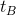## Errors in Einstein's Reasoning (5)

In his paper , Einstein explained the means of clock synchronization this way:

Suppose there are two clocks A and B, so we have two systems of time, called A time and B time respectively. At(A Time), a ray of light was sent out from A to B, and reached B at time(B Time). This ray of light was directly reflected towards A, and reached A at time(A Time). Because in both directions, the light traveled the same distance and at the same speed, thus we can get. This simple equation can be used to synchronize two clocks.

Among the many types of synchronization mechanisms, the one preposed by Einstein is just one special case. As one special case, it has its limitations. For example, it cannot be used when light from one clock can not directly reach the other, or when the two clocks are from different time zones. In our everyday life, the most used method of clock synchronization is one way only.